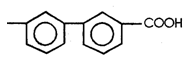Assertion : The difference in the boiling points of equimolar solution of HCI and HF decreases as their

molarity is decreased.

Reason : The extent of dissociation decreases steadily with increasing dilution.

1. If both the assertion and the reason are true and the reason is a correct explanation of the assertion
2. If both the assertion and reason are true but the reason is not a correct explanation of the assertion
3. If the assertion is true but the reason is false
4. If both the assertion and reason are false

Concept Questions :-

Van’t Hoff Factor
High Yielding Test Series + Question Bank - NEET 2020

Difficulty Level:

Assertion : The molar mass obtained for benzoic acid in benzene is found to be nearly

Reason : Benzoic acid has the formula $\mathrm{HOOC}$1. If both the assertion and the reason are true and the reason is a correct explanation of the assertion
2. If both the assertion and reason are true but the reason is not a correct explanation of the assertion
3. If the assertion is true but the reason is false
4. If both the assertion and reason are false

Concept Questions :-

Van’t Hoff Factor
High Yielding Test Series + Question Bank - NEET 2020

Difficulty Level:

Assertion : When 'a' mL of a 0.1 molal urea solution is mixed with another 'b' mL of 0.1 molal glucose

solution, the boiling point of the solution is no different from the boiling points of the

samples prior to mixing but if 'a' mL of 0.1 molal urea is mixed with 'b' mL of 0.1 molal HF

the boiling point of the mixture is different from the boiling points of the separate

samples.

Reason : HF is an electrolyte (weak) whereas glucose is a non electrolyte.

1. If both the assertion and the reason are true and the reason is a correct explanation of the assertion
2. If both the assertion and reason are true but the reason is not a correct explanation of the assertion
3. If the assertion is true but the reason is false
4. If both the assertion and reason are false

Concept Questions :-

Van’t Hoff Factor
High Yielding Test Series + Question Bank - NEET 2020

Difficulty Level:

The latent heat of vaporisation of water is 540 cal ${g}^{-1}$at $100°C$. Kb for water is

Concept Questions :-

Elevation of Boiling Point
High Yielding Test Series + Question Bank - NEET 2020

Difficulty Level:

Assertion :  When a cell is placed in hypertonic solution, it shrinks.

Reason : Reverse osmosis is used for desalination of water.

1. If both the assertion and the reason are true and the reason is a correct explanation of the assertion
2. If both the assertion and reason are true but the reason is not a correct explanation of the assertion
3. If the assertion is true but the reason is false
4. If both the assertion and reason are false

Concept Questions :-

Osmosis and Osmotic Pressure
High Yielding Test Series + Question Bank - NEET 2020

Difficulty Level:

Assertion : Perfectly ideal solution is not possible with respect to binary solution of two liquids.

Reason : No two substances can have exactly the same nature of intermolecular forces & also of the same magnitude.

1. If both the assertion and the reason are true and the reason is a correct explanation of the assertion
2. If both the assertion and reason are true but the reason is not a correct explanation of the assertion
3. If the assertion is true but the reason is false
4. If both the assertion and reason are false

Concept Questions :-

Introduction/ Colligative properties
High Yielding Test Series + Question Bank - NEET 2020

Difficulty Level:

The Van't Hoff factor for 0.1 M $\mathrm{Ba}{\left({\mathrm{NO}}_{3}\right)}_{2}$ solution is 2.74. The degree of dissociation is [IIT 1999]

(1) 91.3%

(2) 87%

(3) 100%

(4) 74%

Concept Questions :-

Van’t Hoff Factor
High Yielding Test Series + Question Bank - NEET 2020

Difficulty Level:

The depression in freezing point of 0.01 M aqueous solutions of urea, sodium chloride and sodium sulphate is in the ratio of [Roorkee 1990; DCE 1994]

(1) 1 : 1 : 1

(2) 1 : 2 : 3

(3) 1 : 2 : 4

(4) 2 : 2 : 3

High Yielding Test Series + Question Bank - NEET 2020

Difficulty Level:

The molal freezing point constant for water is 1.86°C/m. Therefore, the freezing point of 0.1 m NaCl solution in water is expected to be [MLNR 1994]

(1) –1.86°C

(2) –0.186°C

(3) –0.372°C

(4) +0.372°C

Concept Questions :-

Depression of Freezing Point
High Yielding Test Series + Question Bank - NEET 2020

Difficulty Level:

The molar freezing point constant for water is 1.86°C/mole. If 342 g of cane sugar $\left({\mathrm{C}}_{12}{\mathrm{H}}_{22}{\mathrm{O}}_{11}\right)$ is dissolved in 1000 g of water, the solution will freeze at

(1) –1.86°C

(2) 1.86°C

(3) –3.92°C

(4) 2.42°C

Concept Questions :-

Depression of Freezing Point